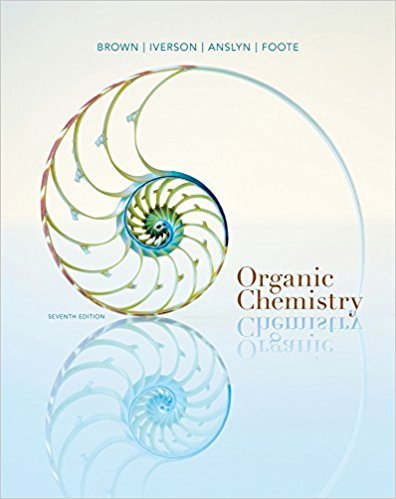×
Get Full Access to Organic Chemistry - 7 Edition - Chapter 2 - Problem 2.21
Get Full Access to Organic Chemistry - 7 Edition - Chapter 2 - Problem 2.21

×

# an !OH (hydroxyl group, Section 1.3A). Which structural formulas represent theISBN: 9781133952848 483

## Solution for problem 2.21 Chapter 2

Organic Chemistry | 7th Edition

• Textbook Solutions
• 2901 Step-by-step solutions solved by professors and subject experts
• Get 24/7 help from StudySoup virtual teaching assistantsOrganic Chemistry | 7th Edition

4 5 1 320 Reviews
21
3
Problem 2.21

an !OH (hydroxyl group, Section 1.3A). Which structural formulas represent the samecompound? Which represent constitutional isomers?

Step-by-Step Solution:
Step 1 of 3

Student: Sarita Peterson October 13, 2015 Lab Partner: Domonique McPhall Chemical Reactions of Copper Objective: The objective of this lab was to understand the law of conservation of mass more in-depth and to become more familiar with the other lab techniques and types of experiments. Introduction: In this experiment copper ribbons will be converted into different stages through the process of many different chemical reactions. These chemical reactions include the disappearance of a solid, the formation of a gas and precipitate, color changes, and temperature changes. At the end of the experiment the copper that is converted back into a solid will be weighed in an attempt to verify the law of conservation of mass. Procedure: We followed the procedures outlined in the course lab manual, CHM 111 General Chemistry Laboratory Manual, LaSalle University, Fall 2015 Experimental Results: Cu = 1.07 g Copper #1- Copper #2- 1.55 g 0.95 g Cu Start = Cu End = 1.00 g 0.95 g Questions: In-Lab Questions: 1. Write the correct name, formula and color for each copper containing compound in this experiment. Describe any observation that accompanied each reaction. a. Name Chemical Formula Observations Copper (II) Nitrate Cu(NO 3 2 When nitric acid was added to the copper powder a brown gas

Step 2 of 3

Step 3 of 3

##### ISBN: 9781133952848

Since the solution to 2.21 from 2 chapter was answered, more than 251 students have viewed the full step-by-step answer. This textbook survival guide was created for the textbook: Organic Chemistry, edition: 7. Organic Chemistry was written by and is associated to the ISBN: 9781133952848. This full solution covers the following key subjects: . This expansive textbook survival guide covers 24 chapters, and 1131 solutions. The answer to “an !OH (hydroxyl group, Section 1.3A). Which structural formulas represent the samecompound? Which represent constitutional isomers?” is broken down into a number of easy to follow steps, and 16 words. The full step-by-step solution to problem: 2.21 from chapter: 2 was answered by , our top Chemistry solution expert on 03/16/18, 05:02PM.

## Discover and learn what students are asking

Calculus: Early Transcendental Functions : Functions of Several Variables
?In Exercises 15 - 22, find all first partial derivatives. $$f(x, y)=\frac{x y}{x+y}$$

Calculus: Early Transcendental Functions : Basic Differentiation Rules and Rates of Change
?Finding a Derivative In Exercises 3–24, use the rules of differentiation to find the derivative of the function. $$f(x)=-9$$

Statistics: Informed Decisions Using Data : Testing the Significance of the Least-Squares Regression Model
?Concrete. As concrete cures, it gains strength. The following data represent the 7-day and 28-day strength (in pounds per square inch) of a certain ty

Unlock Textbook Solution